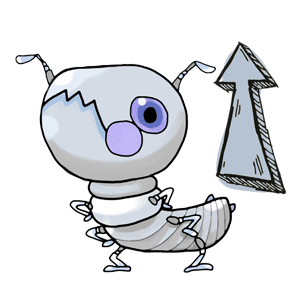# Team:ZJU-China/Modeling/second

## Abstract

In our Termite Application Experiments, we have tested cellulase activity in Coptotermes formosanus alimentary tract. When the activity gets higher, the degradation rate of CNC coat increases. As a result, we must monitor the thickness to prevent the Toxins from leaking so much before trophallaxis takes place. Also, the leaked Toxins must work before our production is excreted.

Fick's first law relates the diffusive flux to the concentration under the assumption of steady state. It postulates that the fluid flows from regions of high concentration to regions of low concentration, which is proportional to the concentration gradient (spatial derivative). In other words, a solute will flow from a high concentration region to a low concentration region across a concentration gradient.

Fick's second law predicts how the diffusion causes the time-varying of concentration.

In our modeling, three processes link to mass transfer, mass transfer in the cell, the CNC-coat transfer in air, the alimentary tract such as mouth, foregut and midgut. Above, the most significant process is the CNC-coat transfer in the alimentary tract.

Fick Law

Step Function

Concentration

## Transmition of Toxin

### Assumptions

We suppose that the transformation rate of CNC coat in the alimentary tract is quite low so that we mainly focus on the transformation rate of Toxin in this model.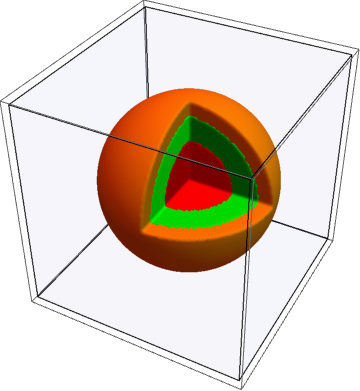Actually, three conditions are different. compared with the transformation rate of that in cell, the transformation rate of CNC coat is lower because the CNC coat is solid while there are mainly colloid in a cell. However, the rate out of CNC coat is higher, because the streaming in alimentary tract provides a higher mass transfer coefficient to the system.

The parameters in this model are qualitative. Without caring about the exact number of the concentration of thickness here, we want to build a base and show the significance of the CNC coat in this static model.

### Equations of Static Model

Based on the Fick law, here are partial differential equations which describe the transformation of Toxin with static CNC coat:

$$\frac{\partial {C_{Toxin}}}{\partial t}={\nabla }({{D}_{Toxin}}{{\nabla }}{C_{Toxin}})$$

In the function, $$C_{Toxin}$$ is the concentration of Toxin, $$t$$ is the time. $$D_{Toxin}$$ is the transformation rate, which differ from the radius because of the different media.

The function is similar to Laplace's equation, but what distinguishes them is that the transformation rate is not a constant. In our assumption, the following figure shows the rate of the radius.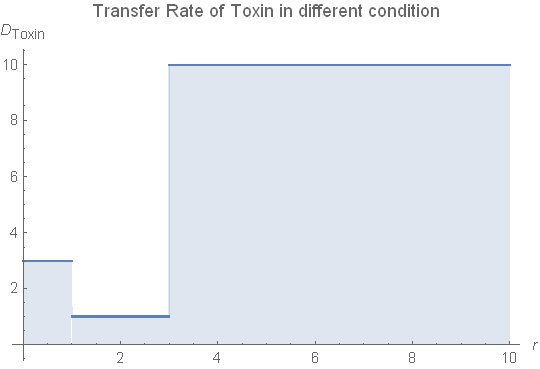In the spherical coordinate system, the gradient of a scalar A is $$\nabla A = \textbf{a}_R \frac\partial{\partial r}(A)$$, the divergence of a position vector A is $$\nabla \cdot\textbf{A} = \frac1{R^2} \frac \partial {\partial R} (R^2A_R)$$,when the system is radially symmetrical. So we get the function in spherical coordinate system:

$$\frac{\partial {C_{Toxin}}(r,t)}{\partial t}=\frac\partial{\partial r}{{D}_{Toxin}}\frac\partial{\partial r}{C_{Toxin}}(r,t)+{{D}_{Toxin}}\frac{\partial^2}{\partial r^2}{C_{Toxin}}(r,t))$$

Given suitable boundary condition, the partial diffusion equation can be solved by Mathematica.

### Boundary Condition

Below is the boundary condition:

$$\begin{eqnarray*} {C_{Toxin}}(r,0)&=&0 \text{ , r}\in \text{(r2,+}\infty \text{)} \\ {C_{Toxin}}(r,0)&=&Constant \text{ , r}\in \text{(0,r2)} \\ \end{eqnarray*}$$

Above, $$r1$$ is the radius of the cell and $$r$$2 is the radius of CNC coat. In fact, $$r2$$ is not a constant, but it follows the normal distribution. We give the assumption $$r2=125nm$$.

First equation ensures that the solution is homogeneous before our production is ate by termites. Supposing the Toxin hardly volatilize into the air, the mass is conservative and the concentration is invariant. In the air, conservation is almost zero.

### Transfer Coefficient

We have discussed the transfer coefficient in section equations for transmiting model, but it is unpractical to use the step function $$u(t)$$to indicate the Transfer Coefficient. Firstly, there is transformation in the cell-CNC coat layer and CNC-coat surface. The coefficient is continuous. Moreover, warnings occur when the PDE function contains step function.

A suitable method to deal with this problem is using the similar continuous function instead of the step function. In our modelling, $$\arctan$$ function is chosen.

Let $$D_1,D_2,D_3$$ be the Transfer Coefficients of Toxin in cell, CNC-coat and the alimentary tract, the Transfer Coefficient $$D_{Toxin}$$ can be present as followed:

$$D_{Toxin} = D_1(u(r)-u(r-r1))+D_2(u(r-r1)-u(r-r2))+D_3u(r-r2)$$

After the optimization, the function $$u(t)$$ is replaced by function $$\arctan(100t)/\pi+0.5$$. The Transfer Coefficient $$D_{Toxin}$$ becomes

$$D_{Toxin} = D_1+\frac{2\arctan(100(r-r1))/+\pi}{2\pi}(D_2-D_1)+\frac{2\arctan(100(r-r2)/+\pi}{2\pi}(D_3-D_2)$$

Followed figures show the difference between two models .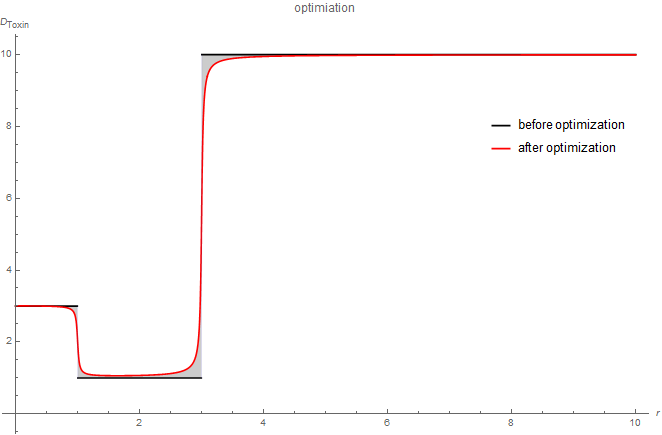### Results

The followed picture shows the result solved by Mathematica. Firstly, the Toxin erodes CNC-coat. Because of the larger radius of the coat, Toxin in the cell decreases with eroding. In the initial state,The transmission speed is higher and it get slow gradually.

Unfortunately, we don't know the exact transmit number for both in cell or CNC-coat. But the cell is liquid, and it is usually $$10$$ times larger than CNC-coat, which suggests that the coat does work.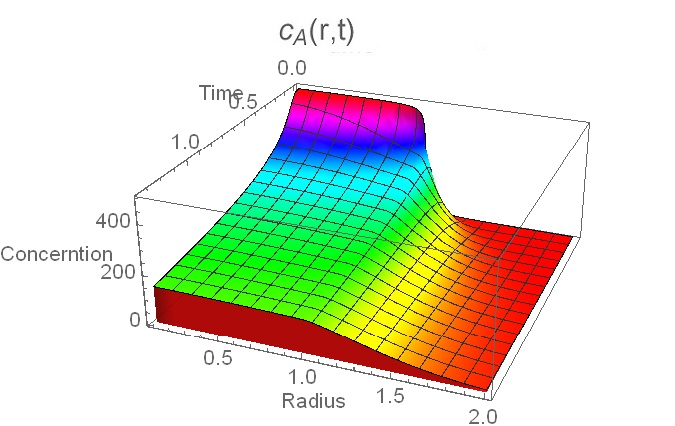### Assumptions

The static model shows the Toxin transmission in or out of a cell with a constant CNC coat. However, the boundary of the CNC coat resets, so we control the outside concentration to kill termites at the suitable time. This part mainly solves the problem of dynamic boundary and discuss how transformation changes for Toxin.

Enzyme Activity is the ability to catalyze a certain chemical reaction. We suppose it can influence the concentration of the reaction product at the boundary when the reaction substrate is plenty.

### Equations for Decomposed Coat

For the cellulose is outside the cell, and in termite's alimentary tract, CNC-coat is digested from its edge. The reaction is $$n(C6)=(C6)_n+(n-1)H_20$$ . Generally, we use Michaelis-Menten equation to evaluate the process.$$v_{C6}=-\frac{V_{max,2}[{C6}]}{K_{m}+[{C6}]}$$$$V_{max,2}$$ is the reducing velocity when C6 is saturated, and $$K_{m}$$ is the Michaelis constant. However,$$[[C6]]$$ is the concerntration of CNC coat, which is solid rather than solution. As a result, we should found another method to evaluate the process. Let's see what happens. Firstly cellulase turn the CNC coat into gucose, the uneven concentration of gucose make gucose spread. Let $$N_A$$ be the voltage of spread. From mass conservation we know $$\nabla N_A =0$$, which is $$\frac{\partial}{\partial r}(r^2 N_{Ar})=0$$, or $$(r^2 N_{Ar})=Constant$$ in the spherical coordinate system.

Once again, let's think about Fick Law, microformat is $$N_{Ar}=-cD_{gucose}\frac{\partial y_A}{\partial r}+y_A N_{Ar}$$, which is equivalent to $$N_{Ar}dr = -\frac{cD_{gucose}}{1-y_A}dy_A$$. Noticed $$(r^2 N_{Ar})=Constanst$$, integral the equation we get the function.$$N_{Ar_0}r_0^2=cD_{gucose}ln(\frac1{1-y_{As}})r$$ ,here $$y_{As}$$ is the volume fraction of gucose in termite's alimentary tract.

The last important conception is about $$N_A$$. For it is the voltage of spread, the mass spread equals to the mass CNC coat reduced, that is, $$4\pi r_0^2 N_{Ar} = -\frac{dV}{dt}\frac{\rho}{M_{CNC}}$$. Here $$N_{Ar}$$ is the spread rate at r-diretion. $$V$$ is the volume of CNC coat, $$\rho$$ and $$M_{CNC}$$ are respectively the density and molecular mass of CNC coat(gucose).

From simultaneous equation of (1) and (2) we get $$\frac{rdr}{dt}\frac{\rho}{M_A} = cD_{gucose}ln(\frac1{1-y_{As}})$$. So we get the relationship between radius and time.

$$R=\sqrt{R_0^2-\frac{2cD_{gucose}}{\rho}M_Aln(\frac1{1-y_{As}})t}=R_0\sqrt{1-kt}$$

Our wetlab members have measured some relative data in this model. Firstly when we put the coat in different part of termites (simulation), the rate is respectively $$0.449U$$, $$1.242U$$, $$1.32716U$$, $$0.947U$$. And $$1U$$ is defines as the micromole of reducing suger producted per minute per milligram protein.

$$the rate = \frac{\partial n_{sugar}}{\partial t}=-\frac{\partial m_{CNC}}{M_{sugar}\partial t}==-\frac{4\pi R^2\rho_{CNC}\partial R}{M_{sugar}\partial t}$$

Known $$\rho_{CNC}=1.5g/cm^3$$( from wikipedia), $$M_{sugar}=180.16g/mol$$(from Baidu pedia) and $$2R=250nm$$(in our dry lab experiment), we get the $$\frac{\partial R}{\partial t}$$ is respectively in a certain condition, the only variable is t. We plot three conditions qualitatively here. The black curve is an Application in the reality. The radius decreases faster when CNC coat is in the midgut and slows down after then. The dotted line is the inner boundary of CNC coat, which disappears when two lines crossed. To simplify the model, we combined the rate in foregut$$(1.242U)$$ and midgut$$(1.327U)$$.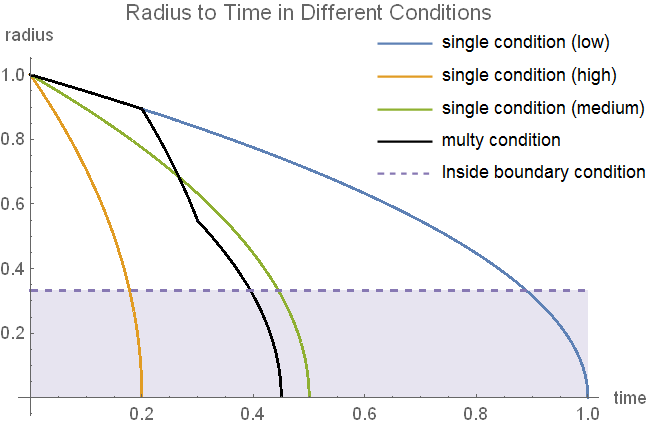### Equations for Dynamic Model

Compared to the static model, the only difference is $$r2$$. Arrange useful equations here:

$$\begin{eqnarray*} \frac{\partial {C_{Toxin}}(r,t)}{\partial t}&=&\frac\partial{\partial r}{{D}_{Toxin}}\frac\partial{\partial r}{C_{Toxin}}(r,t)+{{D}_{Toxin}}\frac{\partial^2}{\partial r^2}{C_{Toxin}}(r,t))\\ {C_{Toxin}}(r,0)&=&C-step(r,r2(0))C \\ D_{Toxin} &=& D_1+step(r,r1)(D_2-D_1)+step(r,r2(t))(D_3-D_2) \\ r(t,D_{gucose} )&=&\sqrt{r2(0)^2-\frac{2cD_{gucose}}{\rho}M_Aln(\frac1{1-y_{As}})t}\\ r2(t)&=&r(t,D_{gucose1})+step(t,t_{gut})((r(t,D_{gucose2})-r(t,D_{gucose1}))-(r(t_{gut},D_{gucose2})-r(t_{gut},D_{gucose1}))) \\ step(x,x0)&=&\frac{2\arctan(100(x-x0))+\pi}{2\pi}\\ \end{eqnarray*}$$

We can conveniently solve the equation array by Mathematica.

### Result

The left figure shows us the transfer rate time-varys. Outside the CNC-coat has the highest rate while the center of the CNC-coat has the lowest. When time is under 0.2 unit, the CNC coat decreases slowly but not linear, but faster when it is in termite's gut. Respectively, the right picture shows how the concentration vary with the transfer rate. Here we can see the CNC-coat can be a good protector that the transformation of Toxin happened only when and after CNC-coat is digested.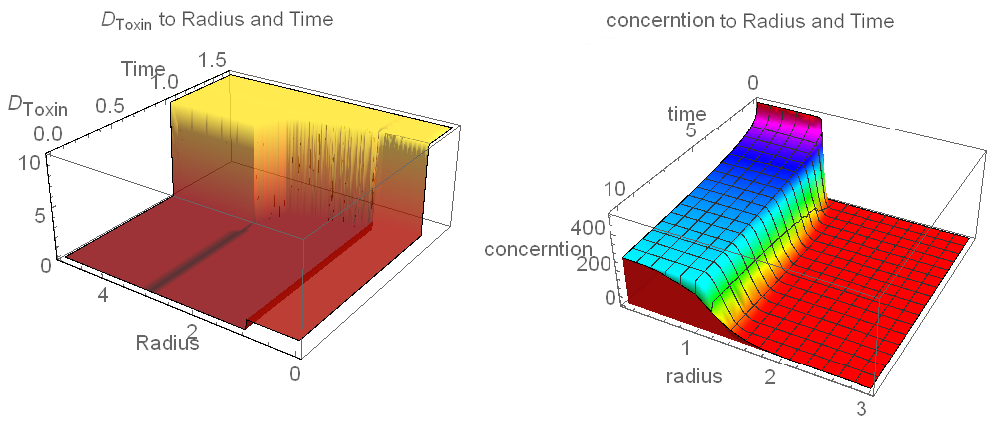## Application

### Overview

Let's see what we have done in the two models. The first model shows us how Toxin acts with a static boundary condition. The point is that Toxin will erode CNC-coat and coat becomes poisonous. Next model shows that how CNC-coat is digested based on our assumption. More importantly, how Toxin acts with the dynamic condition.

The process is as the flowchart below.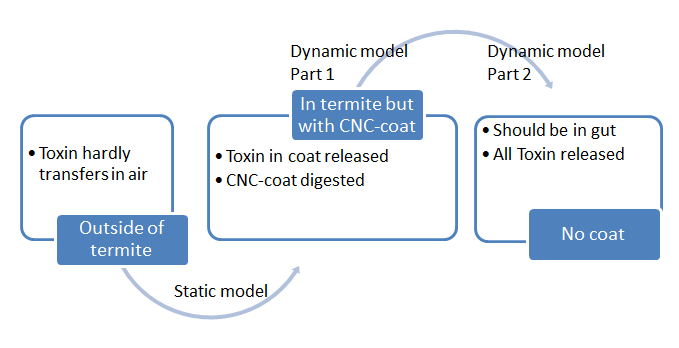### Expiration Date

There is a important time limiting according to our model.

If we leave the production too long, Toxin goes into coat gradually. For the radius is 3-5 times than that of cell, the mass of Toxin in the CNC-coat will be 20 times($$n^3-1)$$ than in the cell. For example, if the coat is digested 0.1 radius in the termite's mouth, the absorbed rateis $$1-0.9^3=0.271$$. That means termites will absorb some Toxin just when they eat our production.

So the Expiration Date is before the Toxin spreads in the whole CNC-coat. Supposing the lethal ratio of the Toxin is $$R_L$$, we have decided the CNC-coat thickness is $$L_t$$. Then we can predict how many Toxin is in the Toxin at a certain time. If the rate begins to grow larger than $$R_L$$, we infer that the production has lost its effect.

### CNC-Coat Thickness

In chemical experiment, we can adjust the thickness of CNC-Coat easily by controlling the concentration of liquid. But how to find the best Thickness that make the best use of the coat?

An achievable method is to do some research about the digestion of CNC. The first part of our dynamic model is to solve this problem. The average time from a termite eats bait to the bait itself comes into another termite's gut(trophallaxis) is usually the same, or service to a certain probability distribution. By the time $$T_{digest}$$ we can choose a suitable thickness that the coat will be completely digested at the same time. Different material has different property, but the function still works.

introduction